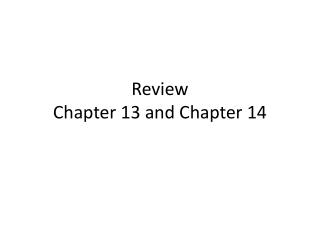DownloadDownload PresentationReview Chapter 13 and Chapter 14

# Review Chapter 13 and Chapter 14

Download Presentation## Review Chapter 13 and Chapter 14

- - - - - - - - - - - - - - - - - - - - - - - - - - - E N D - - - - - - - - - - - - - - - - - - - - - - - - - - -
##### Presentation Transcript

1. Review Chapter 13 and Chapter 14

2. Chapter 13 Outline • Expected Returns and Variances of a portfolio • Announcements, Surprises, and Expected Returns • Risk: Systematic and Unsystematic • Diversification and Portfolio Risk • Systematic Risk and Beta • The Security Market Line (SML)

3. Portfolios • The risk-return trade-off for a portfolio is measured by the portfolio expected return and standard deviation, just as with individual assets Portfolio = a group of assets held by an investor Portfolio weights = Percentage of a portfolio’s total value in a particular asset

4. Portfolio Expected Returns (1) • The expected return of a portfolio is the weighted average of the expected returns for each asset in the portfolio • You can also find the expected return by finding the portfolio return in each possible state and computing the expected value

5. Calculate Portfolio Variance • Portfolio variance can be calculated using the following formula: • Correlation is a statistical measure of how 2 assets move in relation to each other • If the correlation between stocks A and B = -1, what is the standard deviation of the portfolio?

6. Portfolio Diversification

7. Measuring Systematic Risk • Beta (β) is a measure of systematic risk • Interpreting beta: • β = 1 implies the asset has the same systematic risk as the overall market • β < 1 implies the asset has less systematic risk than the overall market • β > 1 implies the asset has more systematic risk than the overall market

8. Reward-to-Risk Ratio: • The reward-to-risk ratio is the slope of the line illustrated in the previous slide • Slope = (E(RA) – Rf) / A • Reward-to-risk ratio = • If an asset has a reward-to-risk ratio = 8? • If an asset has a reward-to-risk ratio = 7?

9. The Fundamental Result • The reward-to-risk ratio must be the same for all assets in the market • If one asset has twice as much systematic risk as another asset, its risk premium is twice as large

10. Security Market Line (2)

11. Chapter 14 Cost of capital and long-term financial policy

12. The Dividend Growth Model Approach • Can be rearranged to solve for RE

13. Example

14. Example

15. Example: Estimating the Dividend Growth Rate • One method for estimating the growth rate is to use the historical average • Year Dividend Percent Change • 20051.03 • 20061.139.7% Geom. Av = 7.97% • 20071.2611.5% • 20081.335.55% • 20091.40 5.26% arithmetic av.=8% • Analysts’ forecast can be used

16. Alternative Approach to Estimating Growth • If the company has a stable ROE, a stable dividend policy and is not planning on raising new external capital, then the following relationship can be used: • A company has a ROE of 17% and payout ratio is 15%. If management is not planning on raising additional external capital, what is its growth rate? Solution: g=17*(1-.15)=14.45% • g = Retention ratio x ROE

17. The SML Approach (CAPM) • Use the following information to compute our cost of equity • Risk-free rate, Rf • Market risk premium, E(RM) – Rf • Systematic risk of asset,  E(RA) = Rf + A(E(RM) – Rf)

18. SML example • Suppose the company has an equity beta of 1.28 and the current risk-free rate is 3.2%. If the expected market risk premium is 9.8%, what is the cost of equity capital? Solution: 15.744%

19. Cost of Equity • Suppose the company has a beta of 1.45. The market return is expected to be 15.2% and the current risk-free rate is 4%. Dividends will grow at 5% per year and last dividend was \$1.2. The stock is currently selling for \$7.35. What is our cost of equity? • Using SML: 20.24% • Using DGM: 22.14%

20. Cost of Debt example • Suppose you have a bond issue currently outstanding that has 17 years left to maturity. The coupon rate is 8% and coupons are paid annually. The bond is currently selling for \$955.874 per \$1000 bond. What is the cost of debt? Solution: 8.5%

21. Cost of Preferred Stock • Preferred stock generally pays a constant dividend every period • Dividends are expected to be paid every period forever • Preferred stock is perpetuity • RP = D / P0

22. Cost of Preferred Stock example • A company has preferred stock that has an annual dividend of \$2. If the current price is \$15, what is the cost of preferred stock? Solution: 13.33%

23. Flotation Costs • The required return depends on the risk, not how the money is raised • However, the cost of issuing new securities should not just be ignored either • Basic Approach • Compute the weighted average flotation cost

24. NPV and Flotation Costs example • A company is considering a project that will cost \$1.2 million. The project will generate after-tax cash flows of \$250,000 per year for 9 years. The WACC is 12% and the firm’s target D/E ratio is .5 (1/2). The flotation cost for equity is 4% and the flotation cost for debt is 2%. What is the average flotation cost? What is the NPV for the project after adjusting for flotation costs?

25. Solution • D=400,000 fd = 400,000*.02 = 8,000 • E=800,000 fE = 800,000*.04 = 32,000 • Fac = .0267 + .0067 =.0334=3.34% • PV=(1,240,000) • PVFCF= 1,332,062.448 • NPV = 92,062.448 positive